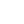# Advent of Code 2022 - Day 3 Javascript Solution

By Henri ParviainenAdvent of Code day 3 problem set has been released! Here is my solution for the day.

## Disclaimer

This solution is not the fastest implementation nor is it claiming to be the best way to solve the problem. There are many ways to solve it and this is just how I did it with Javascript. By sharing I hope it can help someone who is struggling to find a solution to the puzzle.

## Solution

To be able to run my solution, you should have same project setup as me.

First, download the input and save it as a `txt` file in the same directory as you have your javascript files. If you don't yet have any javascript files - make two - one for each part.

So at the starting point, your folder structure should be:

``````/
-- part1.js
-- part2.js
-- input.txt``````

### Part 1

Add the following code to your `part1.js` file.

``````let fs = require("fs");

const backpackItemsList = input.split("\n");

// Helpers

const splitStringFromHalf = (str, index) => {
const result = [str.slice(0, index), str.slice(index)];

return result;
};

const findCommonCharacters = (s1, s2) => {
for (let i in s1) {
let commonChar = s2.includes(s1[i]) ? s1[i] : false;
if (commonChar != "") {
return commonChar;
}
}
};

let countPoints = letter => {
const alpha = Array.from(Array(26)).map((e, i) => i + 65);
const alphabetCapital = alpha.map(x => String.fromCharCode(x));

const alphabetLowercase = alphabetCapital.map(a => a.toLocaleLowerCase());
if (alphabetCapital.includes(letter)) {
const points = alphabetCapital.indexOf(letter, 0) + 27;
return points;
}
if (alphabetLowercase.includes(letter)) {
const points = alphabetLowercase.indexOf(letter, 0) + 1;
return points;
}
};

// Solution for Part 1

let allPoints = 0;

for (const backpack of backpackItemsList) {
let middle = Math.floor(backpack.length / 2);
let [firstCompartment, secondCompartment] = splitStringFromHalf(
backpack,
middle
);

let cc = findCommonCharacters(firstCompartment, secondCompartment);

let points = countPoints(cc);
if (points > 0) {
allPoints = allPoints + points;
}
}

console.log(allPoints);``````

Run in it with `node part1.js` and you'll have your answer for the first part!

### Part 2

For the second part, add the following code to `part2.js`.

``````let fs = require("fs");

const backpackItemsList = input.split("\n");

// Helpers

function findCommonCharacters(s1, s2, s3) {
for (let i in s1) {
let commonChar = s2.includes(s1[i]) && s3.includes(s1[i]) ? s1[i] : false;
if (commonChar != "") {
return commonChar;
}
}
}

let countPoints = letter => {
const alpha = Array.from(Array(26)).map((e, i) => i + 65);
const alphabetCapital = alpha.map(x => String.fromCharCode(x));

const alphabetLowercase = alphabetCapital.map(a => a.toLocaleLowerCase());
if (alphabetCapital.includes(letter)) {
const points = alphabetCapital.indexOf(letter, 0) + 27;
return points;
}
if (alphabetLowercase.includes(letter)) {
const points = alphabetLowercase.indexOf(letter, 0) + 1;
return points;
}
};

// Solution for part 2

const findSumOfAuthenticationBadges = input => {
counter = 0;
points = 0;
let group = [];
for (const backpack of input) {
group.push(backpack);

if (group.length == 3) {
let common = findCommonCharacters(group, group, group);
By running `node part2.js` you have the answer for the second part as well.